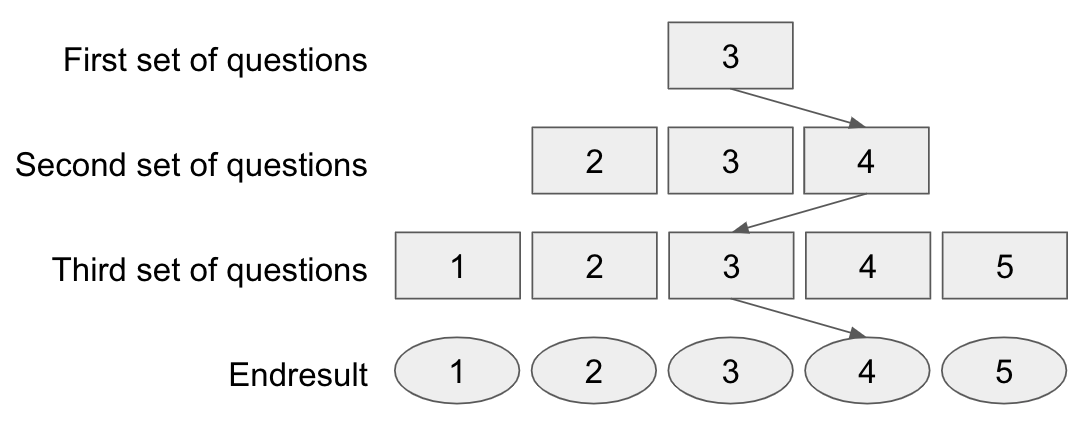# Drillster Support & Info

Creating an adaptive test differs from creating a standard test. You can create an adaptive test by choosing this option in 'Question distribution'. This is an option you can see, when you are creating a new test. Below we explain what to pay attention to when creating an adaptive test. We explain how the starting level works, how a learner goes through the different sets of questions, and we give an example to show how an adaptive test looks.

Start level
If you set up the adaptive test, you will be asked to indicate the start level. With the start level you indicate from which drill Drillster reaches the first set of questions. This is set to "flexible (default)", meaning that Drillster leaves the learner in the middle of level's start.
Level up or down
According to the score a learner achieves on the first set of questions, it continues to the next set of questions. There are 3 possibilities: .
1. The learner answers < ⅓ of the questions correctly, then they drop 1 level.
2. The leaner answers between the  ⅓ & ⅔  of the number of questions correctly, then they remain at the same level.
3. The leaner answers > ⅔ of the questions correctly, then they rise 1 level.
4. When the resulting level is not available (you cannot go lower than the last level or higher than the highest), the learner stays at the same level.

In this way the learner goes through the different levels and eventually the score of the last set of questions determines what score they achieved on the adaptive test.

For example
A student starts the test. For this student it is determined by the managing organization that the starting level is set to 3. The first set of questions comes from the 3rd level.

The student scores 85% in this first set of questions. Because of this score the student ends up in the category >⅔, which makes the student go up one level and thus rises to level 4 in the second set of questions.

In the 2nd set, the student gets a number of questions again. Now 32% is scored correctly. Because of this score, the student ends up in the category <⅓ , which makes the student go down one level and thus goes back to level 3 in the third set of questions.

In the 3rd set the student gets a number of questions again. Here 70% is achieved. Because of this score, the student ends up in the category >⅔ , which makes the student go up one level and thus rises to level 4 again. Because there is no fourth set of questions, the end result of this student is level 4..

Calculation
Just like a normal test, an adaptive test will give a score. Where a normal test can have an outcome of anything between 1% and 100%, the adaptive test can only have a preset number of percentages. For example, when you have an adaptive test with 4 levels, then the score outcome can be: 0%, 25%, 50%, 75% or 100%. The objective of an adaptive test is not to measure the actual knowledge on a specific subject (or the knowledge gap), but to measure on which difficulty level a user masters correctly the specific subject.

To also reflect that indication of what the highest level is that a user masters correctly, there is by default a score transformer when you use an adaptive test, Drill-name with matching level. This transformer makes the link between the test score and the level on which a person masters a topic. For example: if you have 4 drills, named 'drill 1', 'drill 2', 'drill 3', and 'drill 4'. Drill 1 reflects the lowest difficulty level, drill 4 reflects the highest difficulty level.